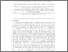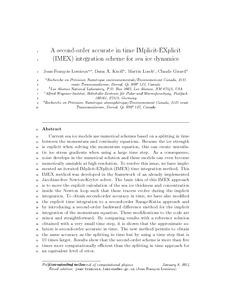### A second-order accurate in time IMplicit–EXplicit (IMEX) integration scheme for sea ice dynamics

Contact
Martin.Losch [ at ] awi.de

Abstract

Current sea ice models use numerical schemes based on a splitting in time between the momentum and continuity equations. Because the ice strength is explicit when solving the momentum equation, this can create unrealistic ice stress gradients when using a large time step. As a consequence, noise develops in the numerical solution and these models can even become numerically unstable at high resolution. To resolve this issue, we have implemented an iterated IMplicit–EXplicit (IMEX) time integration method. This IMEX method was developed in the framework of an already implemented Jacobian-free Newton–Krylov solver. The basic idea of this IMEX approach is to move the explicit calculation of the sea ice thickness and concentration inside the Newton loop such that these tracers evolve during the implicit integration. To obtain second-order accuracy in time, we have also modified the explicit time integration to a second-order Runge–Kutta approach and by introducing a second-order backward difference method for the implicit integration of the momentum equation. These modifications to the code are minor and straightforward. By comparing results with a reference solution obtained with a very small time step, it is shown that the approximate solution is second-order accurate in time. The new method permits to obtain the same accuracy as the splitting in time but by using a time step that is 10 times larger. Results show that the second-order scheme is more than five times more computationally efficient than the splitting in time approach for an equivalent level of error.

Item Type
Article
Authors
Divisions
Primary Division
Programs
Primary Topic
Publication Status
Published
Eprint ID
34895
DOI

Cite as
Lemieux, J. F. , Knoll, D. A. , Losch, M. and Girard, C. (2014): A second-order accurate in time IMplicit–EXplicit (IMEX) integration scheme for sea ice dynamics , Journal of Computational Physics, 263 , pp. 375-392 . doi: 10.1016/j.jcp.2014.01.010Preview
PDF
lemieux-etal_imex.pdf

Cite this document as:

Share

Citation

Research Platforms

Campaigns
N/A

Actions